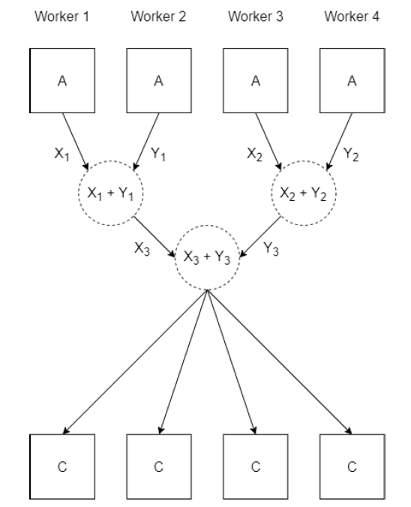# gplus

Add arrays from workers in an `spmd` block

## Syntax

``C = gplus(A)``
``C = gplus(A,destination)``

## Description

example

````C = gplus(A)` add the arrays `A` stored on each worker in the current `spmd` block or communicating job. TipWhen you offload computations using `parfor` and `parfeval`, each computation is run by only one worker at a time. These workers are independent and do not communicate with each other. If you use `gplus` on these workers, then `C` is equal to `A`. The function `gplus` adds the arrays when `A` has a compatible size on each worker.If `numlabs` is equal to `1`, `C` is equal to `A`. `numlabs` is equal to `1` outside of an `spmd` block or communicating job.```
````C = gplus(A,destination)` computes the sum of the arrays and stores the result on only one worker. On the worker with `labindex` equal to `destination`, `C` is equal to the sum. On all other workers, `C` is `[]`.```

## Examples

collapse all

This example shows how to use `gplus` to add a scalar held on each worker in an `spmd` block.

Create a parallel pool with 4 workers.

`parpool(4);`

When you execute an `spmd` block after creating a parallel pool, by default all available workers in the pool will run the code inside the `spmd` block.

Run `labindex` on each worker in the `spmd` block, and store the result in a scalar `A`. Use `gplus` to add the value of `A` from each worker.

When you use `gplus`, by default the result is stored on every worker. On the client, `C` is a `Composite` array. To get the result, index into the `Composite` array.

```spmd A = labindex; C = gplus(A); end disp(C{1})```
` 10`

## Input Arguments

collapse all

Input array, specified as a scalar, vector, matrix, or multidimensional array.

Example: `magic(3)`

Index of target worker, specified as a positive integer scalar. The value must be less than or equal to the value given by `numlabs`, the number of workers running the current `spmd` block or communicating job. When specified, the result of `gplus(A,destination)` is only stored on one worker. On the worker with `labindex` equal to `destination`, `C` is equal to the sum. On all other workers, `C` is `[]`.

Example: `1`

## Algorithms

When you use `gplus(A)`, the value of `A` on each worker is added to the value on other workers.## Version History

Introduced in R2006b